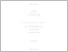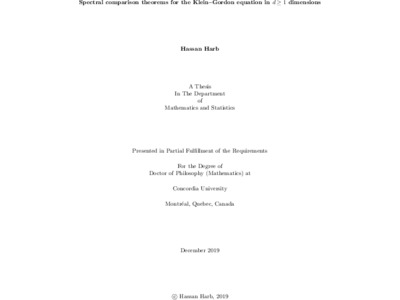Title:

# Spectral Comparison Theorems for the Klein-Gordon equation in d>=1 dimensions

Harb, Hassan (2019) Spectral Comparison Theorems for the Klein-Gordon equation in d>=1 dimensions. PhD thesis, Concordia University.Preview Text (application/pdf) Harb_PhD_S2020 .pdf - Accepted Version 2MB

## Abstract

We first study bound-state solutions of the Klein--Gordon equation φ"(x)=[m^2-〖(E-V(x))〗^2]φ(x), for vector potentials which in one spatial dimension have the form V(x) = vf(x), where f(x)≤0 is the shape of a finite symmetric central potential that is monotone non-decreasing on [0, ∞) and vanishes as x⟶∞, and v>0 is the coupling parameter.

We characterize the graph of spectral functions of the form v= G(E) which represent solutions of the eigen-problem in the coupling parameter v for a given E: they are concave, and at most uni-modal with a maximum near the lower limit E = -m of the energy E ∈ (-m, m). This formulation of the spectral problem immediately extends to central potentials in d > 1 spatial dimensions. Secondly, for each of the dimension cases, d=1 and d ≥ 2, a comparison theorem is proven, to the effect that if two potential shapes are ordered f_1(r) ≤ f_2(r), then so are the corresponding pairs of spectral functions G_1(E) ≤ G_2(E) for each of the existing eigenvalues. These results remove the restriction to positive energies necessitated by earlier comparison theorems for the Klein--Gordon equation by Hall and Aliyu. Corresponding results are obtained when scalar potentials S(x) are also included.

We then weaken the condition for the ground states by proving that if ∫_0^x▒〖[f_2 (t)- f_1 (t)]φ(t)dt≥0〗, the corresponding coupling parameters remain ordered, where φ_i= 1, or φ_i is the bound state solution of the Klein--Gordon equation with potential V_i, i = 1, 2. These results are valid for any energy E ∈ (-m, m)., but they are restricted to the ground states.

We finally present a complete recipe for finding upper and lower spectral bounds for both bounded and unbounded potentials, and we exhibit specific result for the applications for the Woods-Saxon, Gaussian, sech-squared, and Yukawa potentials in dimensions d = 1 and d = 3.

Divisions: Concordia University > Faculty of Arts and Science > Mathematics and Statistics Thesis (PhD) Harb, Hassan Concordia University Ph. D. Mathematics 16 December 2019 Hall, Richard 986526 HASSAN HARB 30 Jun 2021 15:03 01 Jul 2021 01:00
All items in Spectrum are protected by copyright, with all rights reserved. The use of items is governed by Spectrum's terms of access.

Repository Staff Only: item control pageResearch related to the current document (at the CORE website)
Back to top